## RD Sharma Class 8 Solutions Chapter 6 Algebraic Expressions and Identities Ex 6.4

These Solutions are part of RD Sharma Class 8 Solutions. Here we have given RD Sharma Class 8 Solutions Chapter 6 Algebraic Expressions and Identities Ex 6.4

Other Exercises

Find the following products

Question 1.
2a3 (3a + 5b)
Solution:
2a3 (3a + 5b) = 2a3 x 3a + 2a3 x 5b
= 6a3 +1 + 10a3b
= 6a4 + 10a3b

Question 2.
-11a (3a + 2b)
Solution:
-11a (3a + 2b) = -11a x 3a – 11a x 2b
= -33a2– 22ab

Question 3.
-5a (7a – 2b)
Solution:
-5a (7a – 2b) = -5a x 7a- 5a x (-2b)
= -35a2 + 10ab

Question 4.
-11y2 (3y + 7)
Solution:
-11y2 (3y + 7) = -11y2 x 3y – 11y2 x 7
= -33y2+1-77y2
= 33y3-77y2

Question 5.
$$\frac { 6x }{ 5 }$$ (x3+y3)
Solution: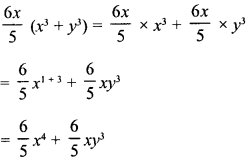Question 6.
xy (x3-y3)
Solution:
xy (x3 – y3) =xy x x3 – xy x y3
= x1 + 3 x y – x x y1+3
= x4y – xy4

Question 7.
0.1y (0.1x5 + 0.1y)
Solution:
0.1y (0.1x5 + 0.1y) = 0.1y x 0.1x5 + 0.1y x 0.1y
= 0.01x5y + 0.01y2

Question 8.Solution: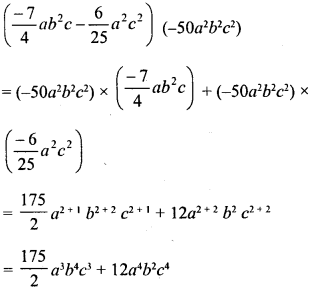Question 9.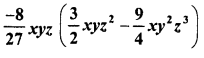Solution:Question 10.Solution: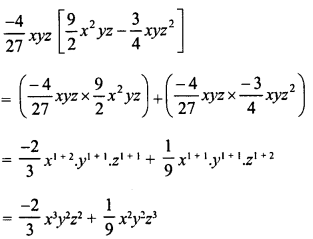Question 11.
5x (10x2y – 100xy2)
Solution:
5x (10x2y – 100xy2)
= 1.5x x 10x2y – 1.5x x 100xy2
= 15x1 + 2y- 150x1+1 x y2
15 x3y- 150xy2

Question 12.
4.1xy (1.1x-y)
Solution:
4.1xy (1.1x-y) = 4.1xy x 1.1x – 4.1xy x y
= 4.51x2y-4.1xy2

Question 13.Solution: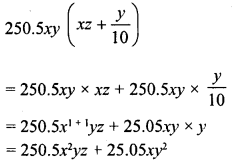Question 14.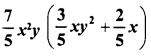Solution: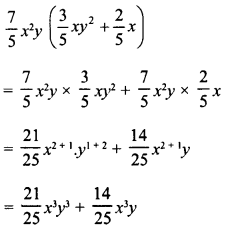Question 15.
$$\frac { 4 }{ 3 }$$ a (a2 + 62 – 3c2)
Solution: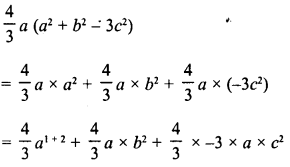Question 16.
Find the product 24x2 (1 – 2x) and evaluate its value for x = 3.
Solution:
24x2 (1 – 2x) = 24x2 x 1 + 24x2 x (-2x)
= 24x2 + (-48x2+1)
= 24x2 – 48x3
If x = 3, then
= 24 (3)2 – 48 (3)3
= 24 x 9-48 x 27 = 216- 1296
= -1080

Question 17.
Find the product of -3y (xy +y2) and find its value for x = 4, and y = 5.
Solution:
-3y (xy + y2) = -3y x xy – 3y x y2
= -3xy2 -3y2 +1  = -3xy2 – 3y3
If x = 4, y = 5, then
= -3 x 4 (5)2 – 3 (5)3 = -12 x 25 – 3 x 125
= -300 – 375 = – 675

Question 18.
Multiply – $$\frac { 3 }{ 2 }$$ x2y3 by (2x-y) and verify the answer for x = 1 and y = 2.
Solution: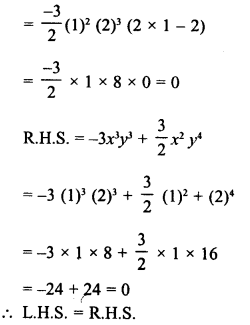Question 19.
Multiply the monomial by the binomial and find the value of each for x = -1, y = 25 and z =05 :
(i) 15y2 (2 – 3x)
(ii) -3x (y2 + z2)
(iii) z2 (x – y)
(iv) xz (x2 + y2)
Solution: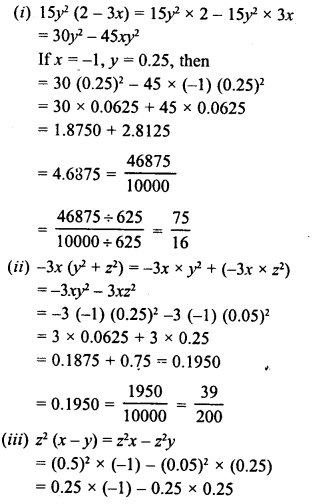Question 20.
Simplify :
(i) 2x2 (at1 – x) – 3x (x4 + 2x) -2 (x4 – 3x2)
(ii) x3y (x2 – 2x) + 2xy (x3 – x4)
(iii) 3a2 + 2 (a + 2) – 3a (2a + 1)
(iv) x (x + 4) + 3x (2x2 – 1) + 4x2 + 4
(v) a (b-c) – b (c – a) – c (a – b)
(vi) a (b – c) + b (c – a) + c (a – b)
(vii) 4ab (a – b) – 6a2 (b – b2) -3b2 (2a2 – a) + 2ab (b-a)
(viii) x2 (x2 + 1) – x3 (x + 1) – x (x3 – x)
(ix) 2a2 + 3a (1 – 2a3) + a (a + 1)
(x) a2 (2a – 1) + 3a + a3 – 8
(xi) $$\frac { 3 }{ 2 }$$-x2 (x2 – 1) + $$\frac { 1 }{4 }$$-x2 (x2 + x) – $$\frac { 3 }{ 4 }$$x (x3 – 1)
(xii) a2b (a – b2) + ab2 (4ab – 2a2) – a3b (1 – 2b)
(xiii )a2b (a3 – a + 1) – ab (a4 – 2a2 + 2a) – b (a3– a2 -1)
Solution:
(i) 2x2 (x3 -x) – 3x (x4 + 2x) -2 (x4 – 3x2)
= 2xx x3-2x2x x-3x x x4-3x x 2x-2x4 + 6x2
= 2x2 + 3– 2x2 +1 – 3x,1+ 4-6x,1+1 -2x4 + 6x2
= 2x5 – 2x3 – 3x5 — 6x2 – 2x4 + 6x2
= 2x5 – 3x5 – 2a4 – 2x3 + 6x2 – 6x2
= -x5 – 2x4 – 2x3 + 0
= -x5-2x4-2x3
(ii) x3y (x2 – 2x) + 2xy (x3 – x4)
= x3y x x2 – x3y x 2x + 2ay x ac3 – 2xy x x4
= x3 + 2y-2x3 + 1 y + 2x1 + 3y – 2yx4+1
= x5y – 2x4y + 2x4y – 2yx5
= -x5y
(iii) 3a2 + 2 (a + 2) – 3a (2a + 1)
= 3a2 + 2a + 4 – 6a2 – 3a
= 3a2 – 6a2 + 2a – 3a + 4
= -3a2 – a + 4
(iv) x (x + 4) + 3x (2x2 – 1) + 4x2 + 4
= x2 + 4x + 3x x 2x2 – 3x x 1 + 4x2 + 4
= x2 + 4x + 6x2 +1 – 3x + 4x2 + 4
= x2 + 4x + 6x3 – 3x + 4x2 + 4
= 6a3 + 4x2 + x2 + 4x – 3x + 4
= 6x3 + 5x2 + x + 4
(v) a (b – c)-b (c – a) – c (a – b)
= ab – ac – be + ab – ac + bc
= 2ab – 2ac
(vi) a (b – c) + b (c – a) + c (a – b)
= ab – ac + bc – ab + ac – bc
= ab – ab + bc – be + ac – ac
= 0
(vii) 4ab (a – b) – 6a2 (b – b2) -3b2 (2a2 – a) + 2ab (b – a)
= 4a2b – 4ab2 – 6a2b + 6a2b2 – 6a2b2 + 3ab2 + 2ab2 – 2a2b
= 4a2b- 6a2b – 2 a2b – 4ab2 + 3 ab2 + 2ab2 + 6a2b2 – 6a2b2
= 4a2b – 8a2b – 4ab2 + 5 ab2 + 0
= – 4a2b + ab2
(viii) x2 (x2 + 1) – x3 (x + 1) – x (x3 – x)
= x2 + 2 + x2 – x3 + 1 – x3 – x1 + 3 + x1 + 1
= x4 + x2-x4-x3-x4 + x2
= x4-x4-x4-x3 + x2 + x2
= -x4 – x3 + 2x2
(ix) 2a2 + 3a (1 – 2a3) + a (a + 1)
= 2a2 + 3 a – 3 a x 2a3 + a2 + a
= 2a2 + 3a – 6a1 + 3 + a2 + a
= 2a2 + 3a – 6a4 + a2 + a
= -6a4 + 3a2 + 4a
(x) a2 (2a – 1) + 3a + a3 – 8
= 2 a2 x a – a2 x 1+3a + a3-8
= 2a3 – a2 + 3a + a3 – 8
= 2a3 + a3 – a2 + 3a – 8
= 3a3 – a2 + 3a – 8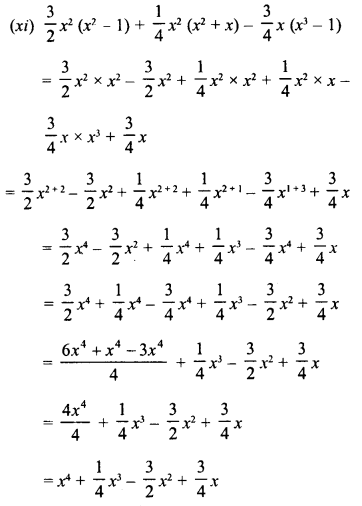(xii) a2b (a – b2) + ab2 (4ab – 2a2) – a3b (1 – 2b)
= a2b x a – a2b x b1 + ab2 x 4ab – ab1 x2a2 -a3b x 1 + a3b x 2b
= a2+1 b-a2b2 +1+ 4a1 +1 b2 +1 -2a2+1 b2-a3b + 2a3b1 +1
= a’b – a2b3 + 4a2b3 – 2a3b2 – a3b + 2a3b2
= a3b – a3b – a2b3 + 4a2b3 – 2a3b2 + 2a3b2
= 0 + 3a2b3 + 0 = 3 a2b3
(xiii) a2b (a3 – a + 1) – ab (a4 – 2a2 + 2a) – b (a3 -a2– 1)
= a2b x a3 – a2b x a + a2b – ab x a2 + ab x 2a2 – ab x 2a- ba3 + ba2 + b
= a2+ 3b – a2+1 b + a2b -a1 + 4b + 2a1 + 2b- 2a1+1 b- a3b + a2b + b
= a5b – a3b + a26 – a5b + 2a3b – 2a2b – a3b + a2b + b
= a5b – a3b + 2a3b – a36 – a3b + a2b – 2a2b + a2b + b
= a3b – a5b + 2a3b – 2a3b + 2a2b-2a2b + b
= 0 + 0 + 0 + b = b

Hope given RD Sharma Class 8 Solutions Chapter 6 Algebraic Expressions and Identities Ex 6.4 are helpful to complete your math homework.

If you have any doubts, please comment below. Learn Insta try to provide online math tutoring for you.